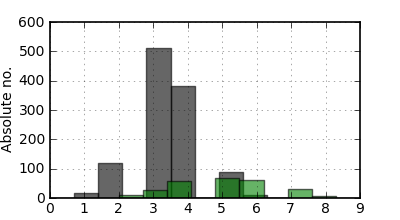# histogram for discrete data

Friends,
I created a histogram plot using data files that have discrete values (sample file attached ‘test.dat’).However when i view the plot, i see that the bars are not located exactly over the values. For example in the attached figure (test.png), i see a bar (gray) placed between values 1 and 2, while there is no such value between 1 and 2. Precisely i would like to know how to make histogram for discrete data values.

The following is my code.

#!/usr/bin/env python
import matplotlib.pyplot as plt
import numpy as np
import matplotlib as mpl
import matplotlib.mlab as mlb

#Creating input file list

#Assignments
FIG=plt.figure(figsize=(4.,2.2),dpi=300)
NROW=1;NCOL=1
mpl.rcParams[‘font.size’]=10
mpl.rcParams[‘lines.linewidth’]=0.8
mpl.rcParams[‘axes.linewidth’]=1.2
mpl.rcParams[‘legend.fontsize’]=10
mpl.rcParams[‘legend.labelspacing’]=0.009

pattern=[‘k’,‘r’]
color=[‘black’,‘green’,‘red’]

for value in range(len(flist)):
n, bins, patches = ax1.hist(data,facecolor=color[value], alpha=0.60,visible=True,histtype=‘bar’,align=‘mid’)
ax1.grid(True,alpha=1.5)
ax1.set_ylabel(‘Absolute no.’,size=10)
plt.savefig(‘test.png’,dpi=100)
ax1.set_xlim([0,9])
plt.show()

test.dat (2.21 KB)···

Bala subramanian, on 2011-02-15 16:06, wrote:

Friends,
I created a histogram plot using data files that have discrete values
(sample file attached 'test.dat').However when i view the plot, i see that
the bars are not located exactly over the values. For example in the
attached figure (test.png), i see a bar (gray) placed between values 1 and
2, while there is no such value between 1 and 2. Precisely i would like to
know how to make histogram for discrete data values.

Hi Bala,

Hist by default will make 10 bins with the same width, so
depending on the distribution of your data, it will shift the
locations of the bins (which is the effect you are seeing).

What you'll want to do is pass the 'bins' keyword to ax.hist to
avoid this. From the docstring

*bins*:
Either an integer number of bins or a sequence giving the
bins. If *bins* is an integer, *bins* + 1 bin edges
will be returned, consistent with :func:`numpy.histogram`
for numpy version >= 1.3, and with the *new* = True argument
in earlier versions. Unequally spaced bins are supported if
*bins* is a sequence.

Here's an example:

In : a = np.random.randint(20,size=(20))

In : ax = plt.gca()

In : ax.hist(a,bins=np.arange(a.min(),a.max()+2,1)-.5)
Out:
(array([1, 3, 1, 1, 0, 3, 1, 0, 0, 0, 0, 2, 1, 0, 1, 1, 0, 0, 2,
3]),
array([ -0.5, 0.5, 1.5, 2.5, 3.5, 4.5, 5.5, 6.5,
7.5,
8.5, 9.5, 10.5, 11.5, 12.5, 13.5, 14.5, 15.5,
16.5,
17.5, 18.5, 19.5]),
<a list of 20 Patch objects>)

In : ax.hist(a) # the default bins=10
(array([4, 2, 3, 1, 0, 2, 1, 2, 0, 5]),
array([ 0. , 1.9, 3.8, 5.7, 7.6, 9.5, 11.4, 13.3,
15.2,
17.1, 19. ]),
<a list of 10 Patch objects>)

best,

···

--
Paul Ivanov
314 address only used for lists, off-list direct email at:
http://pirsquared.org | GPG/PGP key id: 0x0F3E28F7